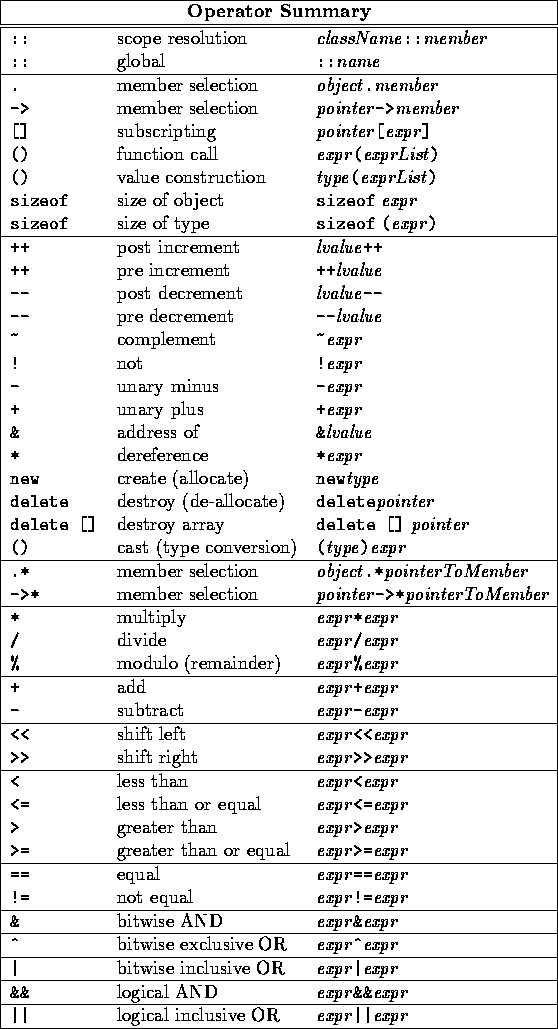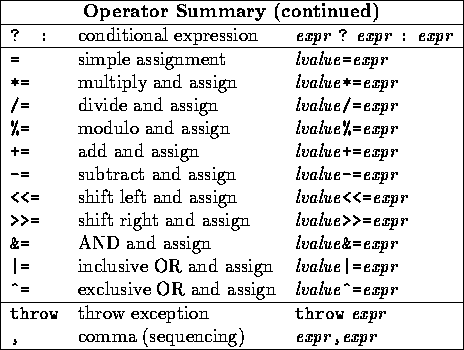# Arithmetic Operators, Expressions, and Precedence

Tom Kelliher, CS17

Feb. 26, 1996

# Arithmetic Operators

Classified by the number of operands

## Unary

+, -, ++, --, ...

```minus_i = -i;
```

Trivia: -1.2

## Binary

+, -, *, /, %

Note:

• % is remainder:
```int number = 456;
int digit0;
int digit1;
int digit2;

digit0 = number % 10;
number = number / 10;
digit1 = number % 10;
number = number / 10;
digit2 = number % 10;
```
Can we use this to get binary?

Can we get the 100's digit without going through the 1's and 10's digits?

# Arithmetic Expressions

Any statement, or part of a statement, with operators:

1. `i = i + 10; // 2 operators`
2. `j++;`
3. `k += 10;`
4. ```while (i <= 10)
;
```

# Order of Evaluation of Arithmetic Expressions

• Parentheses
• Precedence
• Associativity

The entire C++ precedence table:Unary and assignment operators right associate; all others left associate

Precedence, associativity table for the operators we've seen so far:

1. Unary +, - (right)
2. *, /, % (left)
3. Binary +, - (left)
4. Assignment ( =) (right)

Assignment operator:

```i = j = 1000;
```

# Increment and Decrement Operators

• Unary operators
• Pre- and Post-
• Must have a variable as an operand

## Increment

• Pre-Increment: increment the variable, use the new value in the expression
• Post-Increment: use the old value int the expression, increment the variable

Examples:

```int i = 0;
int j = 0;

cout << setw(5) << ++i << j++ << endl;
cout << setw(5) << i << j << endl;
```

A ``side effect:''

```int i;
int j = 10;
i = ++j - 6;   // not good programming practice
```

Something to definitely avoid:

```int i = 0;
i = i++ + ++i;
```

## Decrement

Analogous to increment

# Compound Assignment

Shortcuts for this common situation:

```i = i + 10;
j = j * k;
l = l / (m * 2);
```

Corresponding compound assignments:

```i += 10;
j *= k;
l /= m * 2;
```

Why four ways of adding 1 to a variable?

The compound assignments:

```+=   -=   *=   /=   %=
```

Modified precedence, associativity table for the operators we've seen so far:

1. Unary +, -, ++, -- (right)
2. *, /, % (left)
3. Binary +, - (left)
4. Assignment =, +=, -=, *=, /=, %= (right)

# Practice Problems

Evaluate the following expressions:

```int a = 4;
int b = 5;
int c = 2;
int x = 10;
int y = 3;
int z = 5;

a % b - 5;   // these look funny, but they're valid
c - b * a;
c * c + b * a / 3;
a - b - c * a % b;
x % y * z;
x++ % --y + 7 / z;
((x - 6) * (x - 6) + y * y) / (z * z);
x += y + z;
```

Thomas P. Kelliher
Sat Feb 24 17:23:53 EST 1996
Tom Kelliher